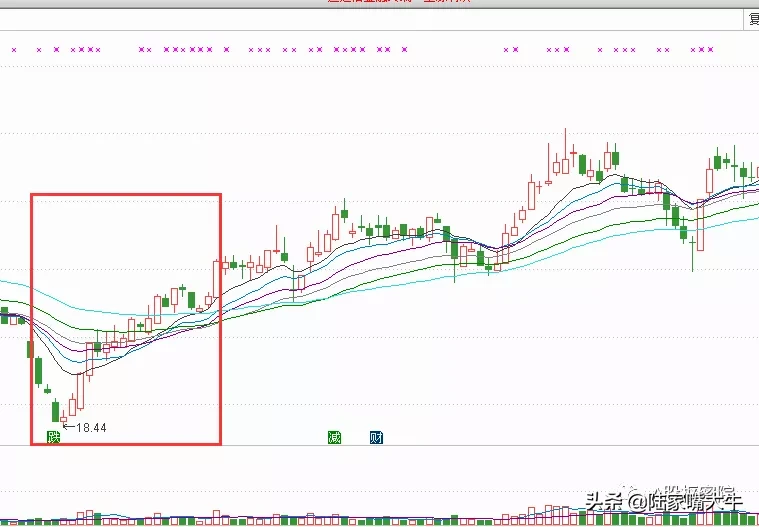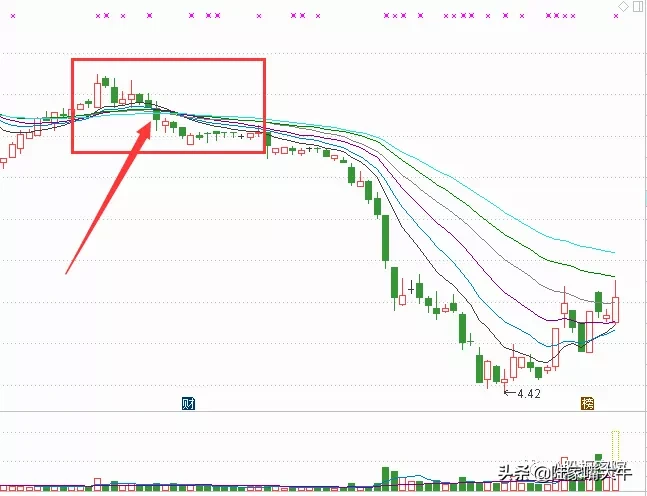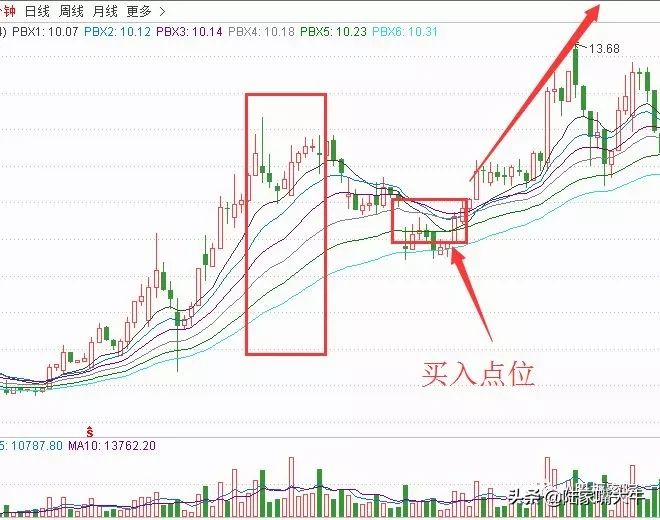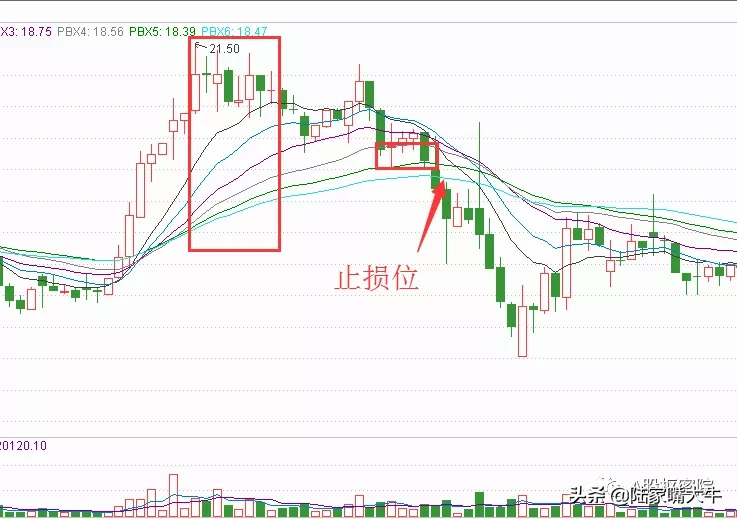# 什么是瀑布线（善用瀑布线指标，巧抓股市主升浪）

PBX1:(EMA(CLOSE,M1)+MA(CLOSE,M1*2)+MA(CLOSE,M1*4))/3;

PBX2:(EMA(CLOSE,M2)+MA(CLOSE,M2*2)+MA(CLOSE,M2*4))/3;

PBX3:(EMA(CLOSE,M3)+MA(CLOSE,M3*2)+MA(CLOSE,M3*4))/3;

PBX4:(EMA(CLOSE,M4)+MA(CLOSE,M4*2)+MA(CLOSE,M4*4))/3;

PBX5:(EMA(CLOSE,M5)+MA(CLOSE,M5*2)+MA(CLOSE,M5*4))/3;

PBX6:(EMA(CLOSE,M6)+MA(CLOSE,M6*2)+MA(CLOSE,M6*4))/3;

1、入场信号

6条瀑布线如果在股票价格处于低位时粘合，短周期瀑布线上穿长周期瀑布线，6条瀑布线同时往上发散。

2、退场信号

6条瀑布线如果在股票价格牌高位时粘合，短周期瀑布线下穿长周期瀑布线，6条瀑布线同时往下发散。

3、持仓信号

6条瀑布线按照从下往上的排列方式排列，并形成多头排列。